# a. Determi n Ma Touries tan form edthe pubeunchin g t) gien by 00 1 <lgl...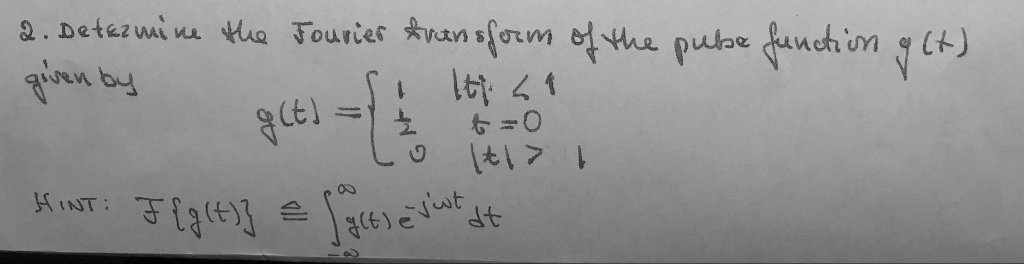a. Determi n Ma Touries tan form edthe pubeunchin g t) gien by 00 1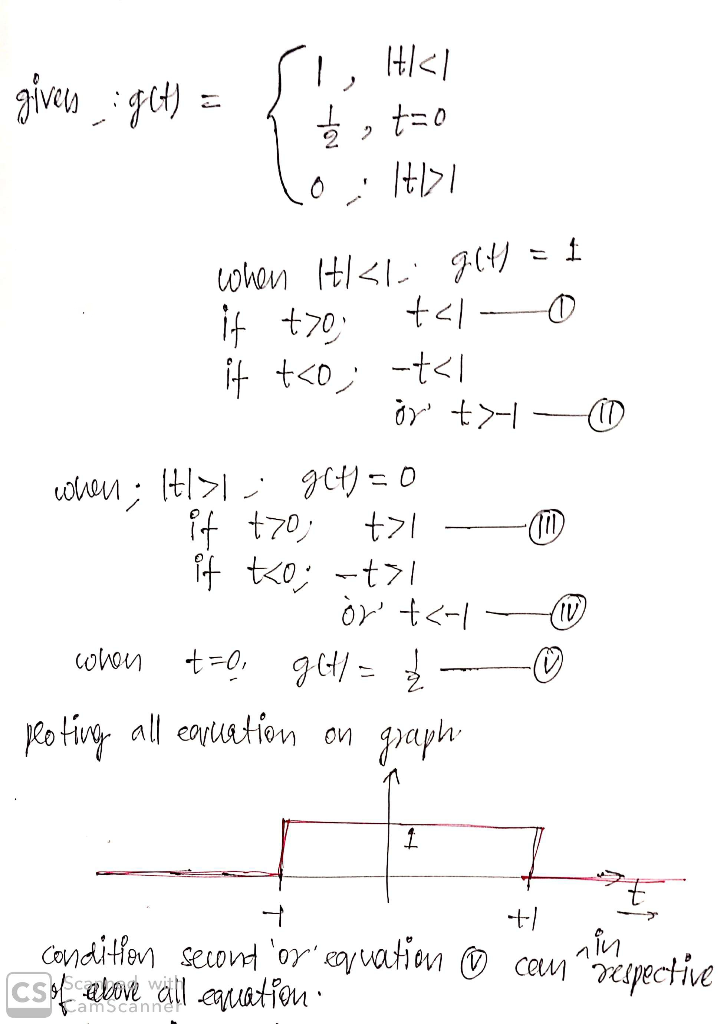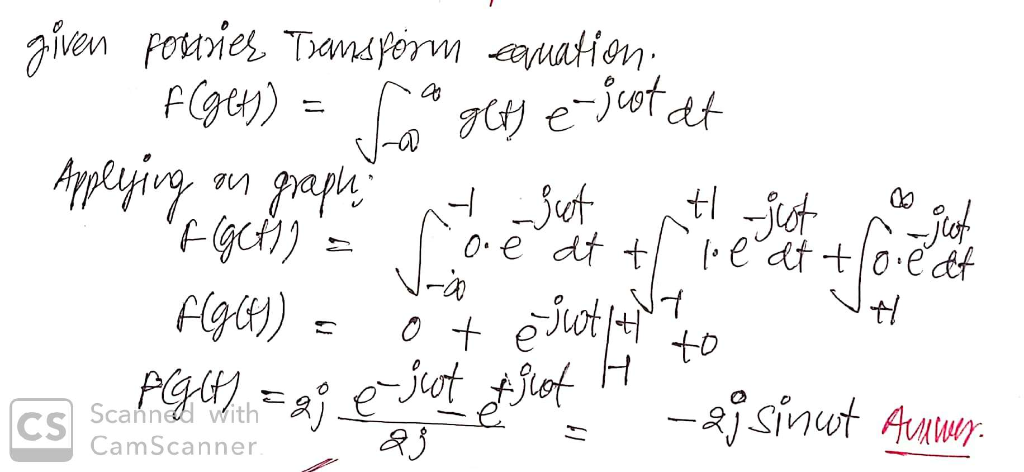##### Add Answer of: a. Determi n Ma Touries tan form edthe pubeunchin g t) gien by 00 1 <lgl...
Similar Homework Help Questions
• ### t, - 1-4, MA 207 t, - 1-4, MA 207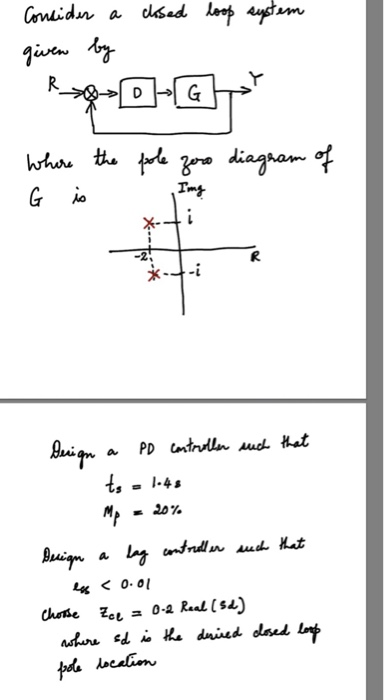t, - 1-4, MA 207 t, - 1-4, MA 207

• ### How does the derivative of f(t) = t(tan(t) equal tan(t)+t(1+tan(t)^2) ?

A question asks to differentiate f(t) = t(tan(t)Answer key gives: tan(t)+t*(1+tan(t)^2)Please explain how to arrive at that answer; what rules of derivatives are used?I use the product rule and I arrive at t(sec^2t) + tan(t)(1)is this not correct?Does sec^2tequal(1+(tan(t))^2) perhaps?Therefore would have have:t * ( 1+tan^2(t) )+ tan(t)??Thanks

• ### 1. (14pts) Circle whether the following limit is an “Indeterminate form” (I) or “Determinate form” (D)...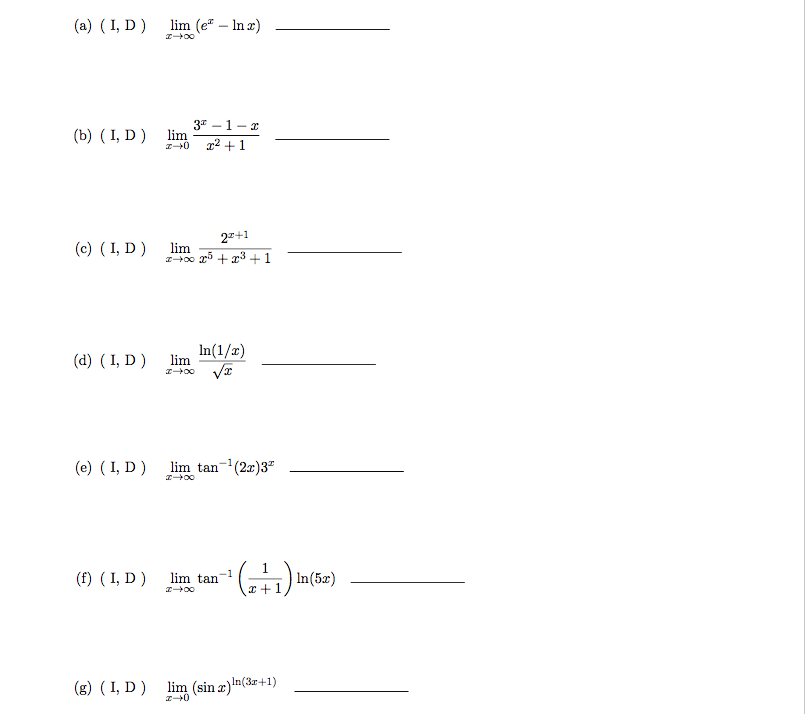1. (14pts) Circle whether the following limit is an “Indeterminate form” (I) or “Determinate form” (D) andstate the type of an inderterminate form (0, 1, 0 · 1, 1 1, 00, 10, and 11) if the limit is an indeterminate form. 0 1 (a) I, D) lim (e -n 3-1-r (b) , D) lm 2 -0 2+1 (c) (I, D) lim o0 1 In(1/2) V (d) I, D) lim (e) (I, D (2:r)3 -1 lim tan (f) (I, D) n(5)...

• ### Problem 1 Suppose there is a series of cashflows that lasts n + 1 peri- ods, {at}t, 0 < t <n, and that is growing...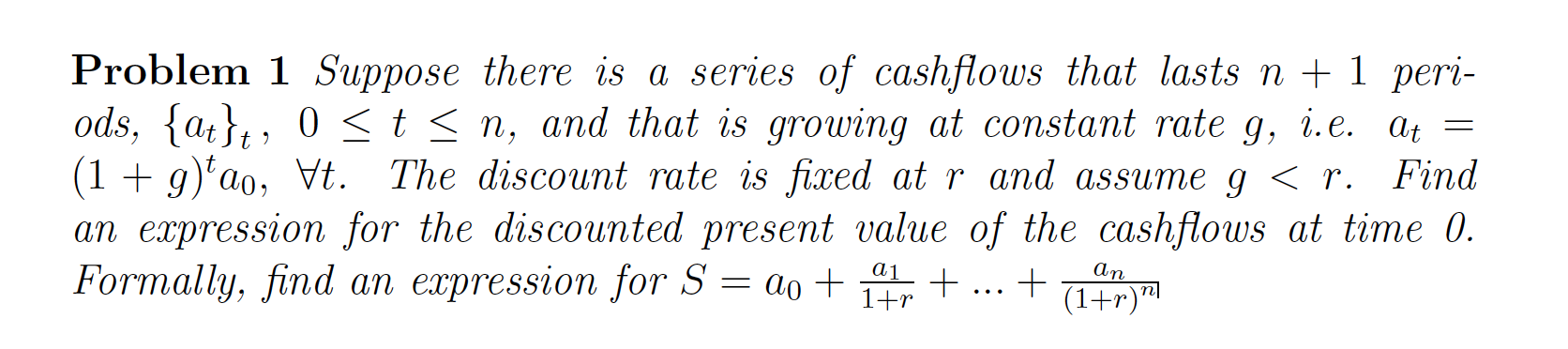Problem 1 Suppose there is a series of cashflows that lasts n + 1 peri- ods, {at}t, 0 < t <n, and that is growing at constant rate g, i.e. At = (1 + g)tao, Vt. The discount rate is fixed at r and assume g < r. Find an expression for the discounted present value of the cashflows at time 0. Formally, find an expression for S = 20 + 141 + ... + (147) ma al αη 1+r

• ### Because f(t)= sin t and g(t)= tan t are odd functions, what can be said about the function h(t)=f(t)g(t), explain

Because f(t)= sin t and g(t)= tan t are odd functions, what can be said about the function h(t)=f(t)g(t), explain

• ### Q9 (Approximation of π) (a) Show that 1/1 + t2 = 1 − t2 + t4 − ... + (−1)n−1 t 2n−2 + (−1)n t2n /1 + t2 for all t ∈ R and n ∈ N. (b) Integrate both side in (a), show that tan−1 (x) = x − x3/3 + x5 /5...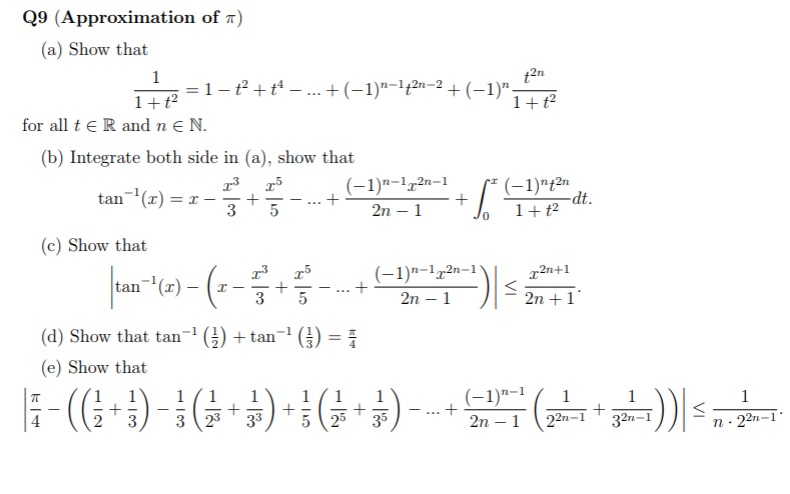Q9 (Approximation of π) (a) Show that 1/1 + t2 = 1 − t2 + t4 − ... + (−1)n−1 t 2n−2 + (−1)n t2n /1 + t2 for all t ∈ R and n ∈ N. (b) Integrate both side in (a), show that tan−1 (x) = x − x3/3 + x5 /5 − ... + (−1)n−1x 2n−1/ 2n − 1 + Z x 0 (−1)n t2n /1 + t2  dt. (c) Show that tan−1 (x) − ( x...

• ### Prove that tan t = ________ ?

Prove that tan t = √1-cos2tcostwhere0 < t < π

• ### Prob. 5 (a) Let x(t) = u(t) and h(t) = e-looor u(t) + e-lotu(t). -00 <t< oo using graphical convolution(s). Determine y(t) = h(t) * x(t) for Prob. 5 (cont.) (b) Let zln] = uln] and h[n]-G)...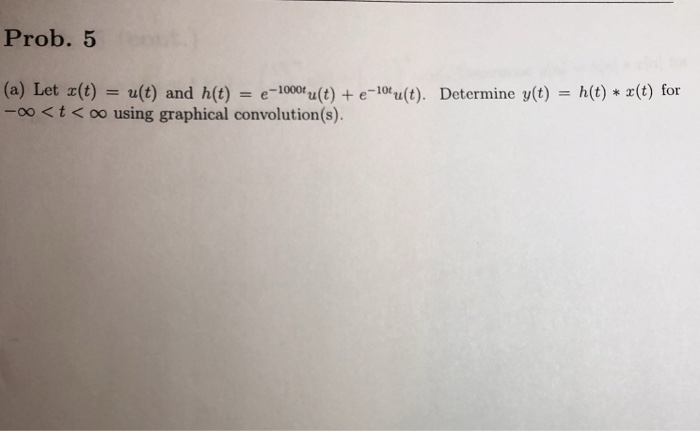Prob. 5 (a) Let x(t) = u(t) and h(t) = e-looor u(t) + e-lotu(t). -00 <t< oo using graphical convolution(s). Determine y(t) = h(t) * x(t) for Prob. 5 (cont.) (b) Let zln] = uln] and h[n]-G)nuln] + (-))' hnnDetermine vinl -h) rin) for -00n< oo using graphical convolution(s) Prob. 5 (a) Let x(t) = u(t) and h(t) = e-looor u(t) + e-lotu(t). -00

• ### r'(t)=sec(t)tan(t)-1, p(0,0)

fin the function with the given derivative whose graph passes through the point P.r'(t)=sec(t)tan(t)-1, p(0,0)

• ### (1 point) Consider the initial value problem y"36y g(t), y(0) 0, /(0) 0, t if 0<t<5 0 if 5t<00. where g(t)...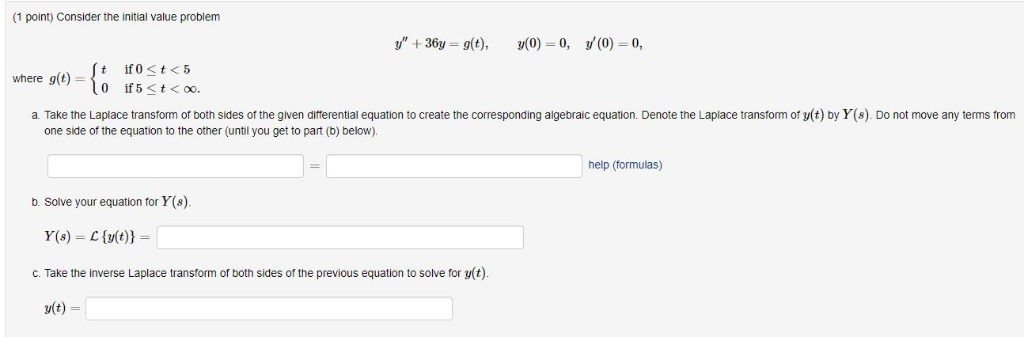(1 point) Consider the initial value problem y"36y g(t), y(0) 0, /(0) 0, t if 0<t<5 0 if 5t<00. where g(t) create the corresponding algebraic equation. Denote the Laplace transform of y(t) by Y(s). Do not move any terms from a. Take the Laplace transform of both sides the given differential equation one side of the equation the other (until you get to part (b) below). help (formulas) b. Solve your equation for Y(s). Y(s) C{y(t)} solve for y(t) c....

Need Online Homework Help?